# Math in Focus Grade 1 Chapter 7 Practice 2 Answer Key Place Value

This handy Math in Focus Grade 1 Workbook Answer Key Chapter 7 Practice 2 Place Value detailed solutions for the textbook questions.

## Math in Focus Grade 1 Chapter 7 Practice 2 Answer Key Place Value

Look at the pictures. Fill in the blanks.

Example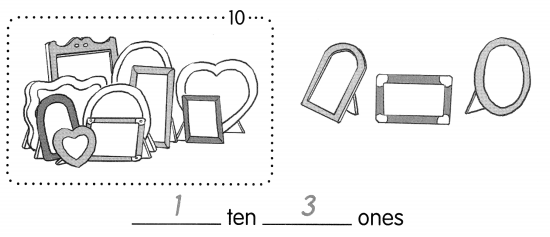Question 1.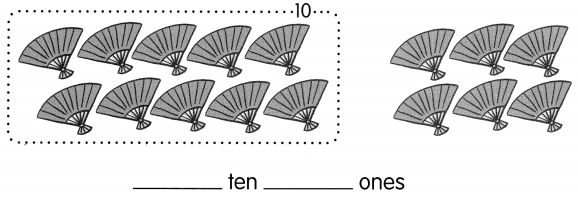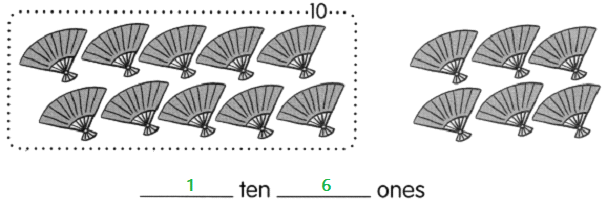Question 2.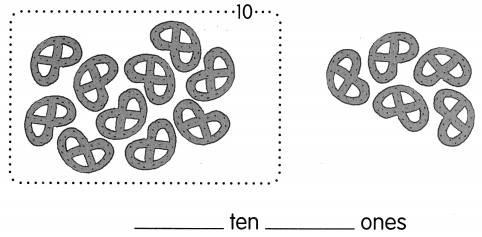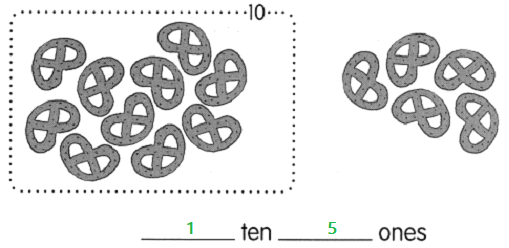Question 3.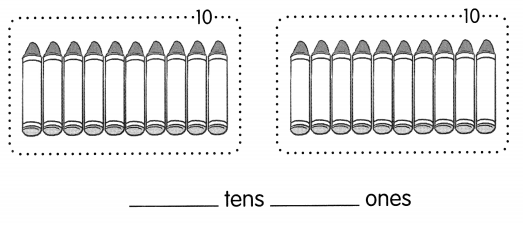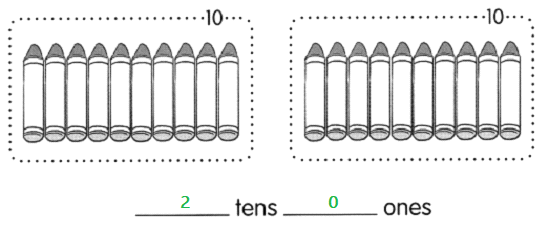Fill in the place-value charts.

Example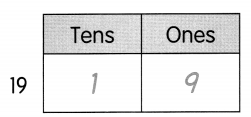Question 4.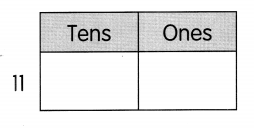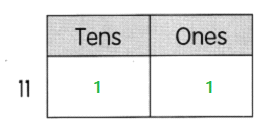Question 5.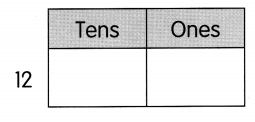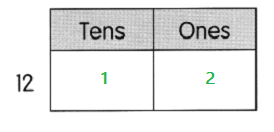Question 6.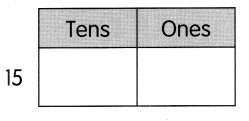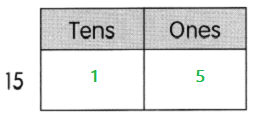Question 7.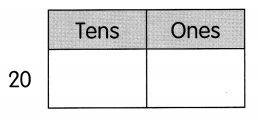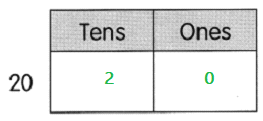Show the number.
Draw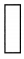for tens and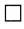for ones.

Example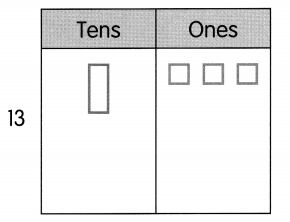Question 8.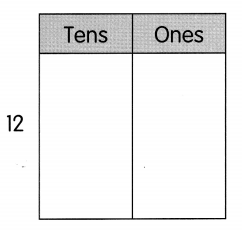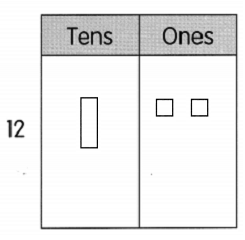Question 9.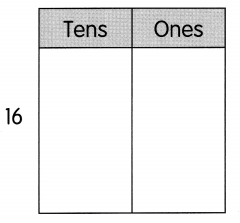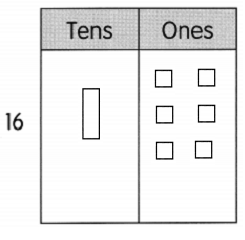Question 10.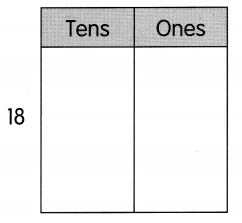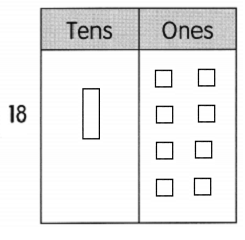Question 11.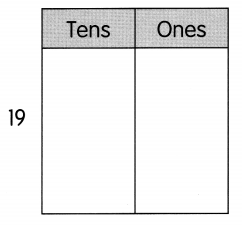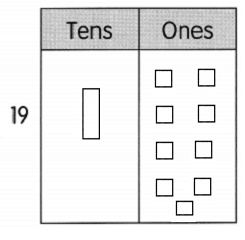Look at the place-value charts. Write the numbers. Fill in the blanks.

Question 12.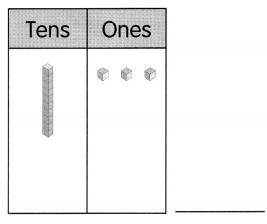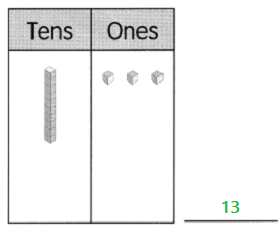Question 13.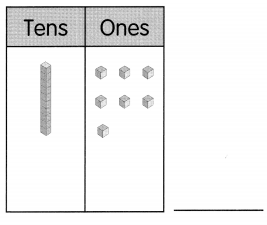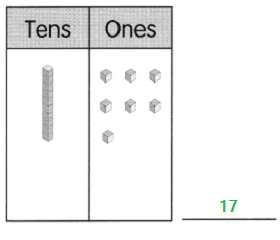Question 14.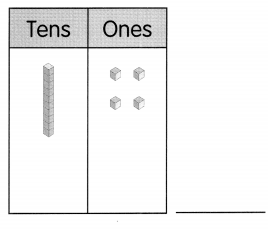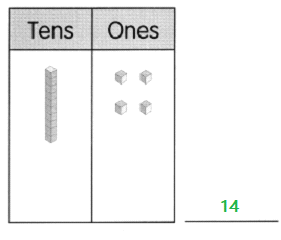Question 15.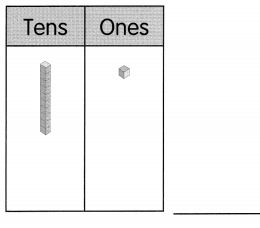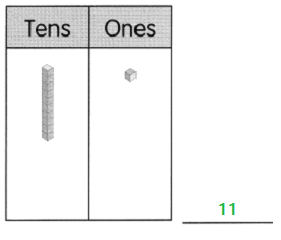Fill in the blanks.

Question 16.
13 = 1 ten ___ ones
Answer: 13 = 1 ten 3 ones

Question 17.
17 = ___ ten 7 ones
Answer: 17 = 1 ten 7 ones

Question 18.
15 = 1 ten ___ ones
Answer: 15 = 1 ten 5 ones

Question 19.
12 = ____ ten 2 ones
Answer: 12 = 1 ten 2 ones

Question 20.
19 = 1 ten ___ ones
Answer: 19 = 1 ten 9 ones